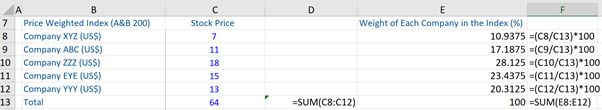## What is a “Price Weighted Index”?

A price-weighted index (PWI) is a type of stock market index where the weighting of each individual stock within the index is equal to the stock price of each individual constituent company divided by the sum of the stock prices of all the companies within the index. In other words, each component of a PWI is weighted according to its current stock price. In such an index, stocks with higher prices (e.g. \$150 per share) are accorded higher weights than stocks with lower prices (\$12 per share). The index weighting does not consider an individual company’s size or the number of shares it has outstanding. Well-known examples of price-weighted indices are the Dow Jones Industrial Average (DJIA) and Japan’s Nikkei 225.

Consequently in a PWI, the stocks with higher prices have a greater impact on the movements or changes in the index than the stocks with lower prices. Therefore, if one of the higher-priced stocks within the index has a large price increase, the index is more likely to increase even if the other stocks in the index with the lower prices decline in value at the same time.

Currently, price-weighted indices are not very common. Instead, the most common type of stock market index is the market value or capitalization-weighted index.

## Key Learning Points

• In a price-weighted index, stocks with higher prices are accorded a higher weighting than stocks with lower prices
• In reality, the calculation of the value of a PWI (for example, the DJIA) involves using a divisor (dow divisor), which is an arbitrary value that is calculated by the index
• The PWI is much less commonly used today, due to certain limitations

## Price Weighted Index – Weights, Divisor, and Limitations

In a PWI, the weight of a constituent company (i.e. included in the index) is computed by dividing its stock price by the sum of all the constituent companies’ stock prices.

Weight = SP1/(SPI + SP2 +… + Sn)

SP = Stock price of each constituent company of the PWI.

For example, if there are five companies included in a PWI, then the weight of company A in the PWI is calculated by dividing its stock price by the sum of the stock prices of all the five companies in the index.

To calculate the value of a PWI, one needs to take an arithmetic average by dividing the total sum of the stock prices of the constituent companies included in the index by the number of the constituent companies included in the index. In theory, this is the way it can be calculated. However, in practice, the value of a PWI is calculated by adding up the stock prices of all the constituent companies that are included in this index and dividing the same by the divisor (which is an arbitrary value that is calculated by the index) and adjusted for varied structural changes in the constituent companies of the index.

For example, in practice, the calculation of the DJIA value is quite complex. It is arrived at by adding up stock prices of the 30 blue-chip companies that are included in this index and then dividing the total value obtained by a number referred to as the dow divisor. This divisor varies over time and is adjusted periodically, in order to reflect changes in the stocks that constitute the 30 blue-chip DJIA and maintain the continuity of this index.

Due to certain limitations, the PWI is much less popular than the market capitalization-weighted index. For example, while a PWI reflects a change in stock prices, it is not indicative of any changes in the stock market. Further, bigger companies with low stock prices will have smaller weightage in such an index, while small companies with higher stock prices may have greater weightage in the same. As a result, a PWI will not show a clear or definitive picture of the stock market. Moreover, the PWI is biased towards high price stocks.

## Calculating Weights of a Price-Weighted Index

Given below is an example of a price-weighted index (we’ve called it the A&B 200). It includes stocks from the 5 constituent companies. The information about these constituent companies and their respective stock prices is given below and subsequent index weightings are calculated.

In this index, what matters is the absolute price of a company’s stock. Company ZZZ has the highest weight (28.1%) in the index, as its stock price is \$18 (highest among other companies in this index). The stock of this company will have a greater impact on the movements or changes in this index, than stocks with lower prices.